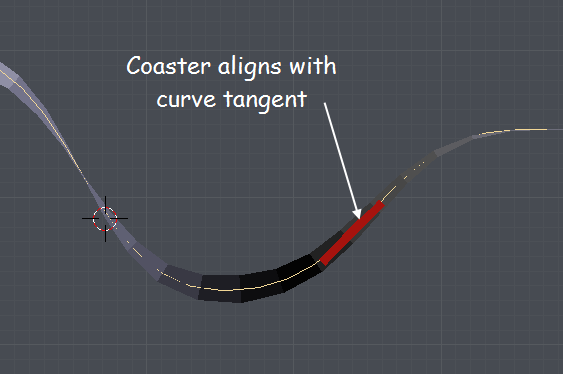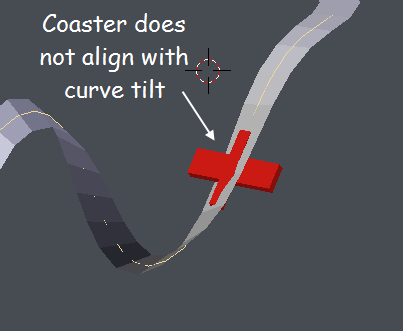# Trying to simulate Follow Path constraint using just python

(BigEfrom84) #1

I am working on a roller coaster project using just python to create the animation. Based on work by Jeremy Behreandt here https://medium.com/@behreajj/scripting-curves-in-blender-with-python-c487097efd13 i have got the coaster to follow the path and align with the curve tangent. My problem lies in not being able to figure out how to rotate the coaster to account for the tilt of the curveBut i can’t figure out how to align coaster to tilt of curveI have attached the blend file

PythonAnimation03.blend (507.1 KB)

(ajcdfin) #2

Hey, I don’t know much coding but I think it has to do with the “Follow” check box under curve > “Path Animation”, but that is just a guess. Also why is your ‘rotation’ space set to ‘Quaternion’ ?

(BigEfrom84) #3

I have the tangent vector at some point on the curve.
tangent = coord_tangent[‘tangent’]
tangent.normalize()
then i align the object to the curve with the following
rotation = tangent.to_track_quat(‘X’, ‘Z’)
object.rotation_quaternion = rotation
… works fine this far …
tilt = … known

What i am looking for is how to apply an additional rotation to get the object to account for the tilt of the curve at that point. I know the tilt angle around the tangent vector.

#4

Hi @BigEfrom84,

Maybe have `bezier_multi_seg` return not just the bezier coordinate and tangent, but the local right, and up for that point on the curve also? I added a helper function that normalizes the tangent and finds the right vector relative to a reference / world up. The right vector is rotated by the tilt around the forward axis. Last, the local up vector is found with the cross product.

``````def axes(tangent, tilt=0.0, ref_up=Vector((0.0, 0.0, 1.0))):
tan_norm = tangent.normalized()
right = tan_norm.cross(ref_up)
rot = Quaternion(tangent, tilt)
right = rot * right
right.normalize()
up = right.cross(tangent)
up.normalize()
return {'right': right, 'up': up, 'forward': tan_norm}
``````

To update the relevant bit of the `bezier_multi_seg` function:

``````def bezier_multi_seg(knots=[], step=0.0, closed_loop=False,
ref_up=Vector((0.0, 0.0, 1.0))):
#...
tangent = bezier_tangent(pt0=pt0, pt1=pt1, pt2=pt2, pt3=pt3, step=u)
tilt = (1.0 - u) * a.tilt + u * b.tilt
ax = axes(tangent, tilt, ref_up)

return {'coord': coord,
'right': ax['right'],
'forward': ax['forward'],
'up': ax['up']}
``````

Then you could build a quaternion from the three axes.

``````    i = coord_tangent['right']
j = coord_tangent['forward']
k = coord_tangent['up']

rotation = Matrix([
[i.x, j.x, k.x, 0.0],
[i.y, j.y, k.y, 0.0],
[i.z, j.z, k.z, 0.0],
[0.0, 0.0, 0.0, 1.0]
]).to_quaternion()
coaster.rotation_quaternion = rotation
``````

Sorry, I can’t upload a .blend file attachment atm, otherwise I would.